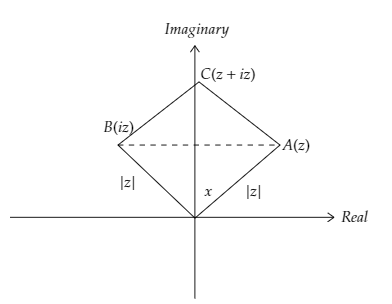Courses
Courses for Kids
Free study material
Free LIVE classes
More# What is the area of the triangle with vertices $A\left( z \right)$, $B\left( {iz} \right)$, and $C\left( {z + iz} \right)$?A. $\dfrac{1}{2}{\left| {z + iz} \right|^2}$B. 1C. $\dfrac{1}{2}$D. $\dfrac{1}{2}{\left| z \right|^2}$Verified
91.2k+ views
Hint: The angle between vertex $A\left( z \right)$ and $B\left( {iz} \right)$ is ${90^ \circ }$. So, the base of the triangle is $\left| z \right|$ and the height of the triangle is $\left| {iz} \right|$. By using the area formula of a triangle, we can calculate the area of the $\Delta ABC$.

Formula Used:
The angle between two complex numbers ${z_1} = {x_1} + i{y_1}$ and ${z_2} = {x_2} + i{y_2}$ is $\theta = {\tan ^{ - 1}}\left( {\dfrac{{\dfrac{{{y_1}}}{{{x_1}}} - \dfrac{{{y_2}}}{{{x_2}}}}}{{1 + \dfrac{{{y_1}}}{{{x_1}}} \cdot \dfrac{{{y_2}}}{{{x_2}}}}}} \right)$.
The modulus of complex number $z = x + iy$ is $\left| z \right| = \sqrt {{x^2} + {y^2}}$
Area of a triangle$= \dfrac{1}{2} \times {\rm{base}} \times {\rm{height}}$

Complete step by step solution:
Given that the vertices of the triangle are $A\left( z \right)$, $B\left( {iz} \right)$, and $C\left( {z + iz} \right)$.Image: Triangle ABC
Now putting $z = x + iy$ in vertices
The vertices are $A\left( {x + iy} \right)$, $B\left( {i\left[ {x + iy} \right]} \right)$, and $C\left( {x + iy + i\left[ {x + iy} \right]} \right)$ or $A\left( {x + iy} \right)$, $B\left( { - y + ix} \right)$, and $C\left( {x - y + iy + ix} \right)$. $\because i^{2}=-1$
Now applying the formula $\theta = {\tan ^{ - 1}}\left( {\dfrac{{\dfrac{{{y_1}}}{{{x_1}}} - \dfrac{{{y_2}}}{{{x_2}}}}}{{1 + \dfrac{{{y_1}}}{{{x_1}}} \cdot \dfrac{{{y_2}}}{{{x_2}}}}}} \right)$ to calculate the angle between $A\left( {x + iy} \right)$ and $B\left( { - y + ix} \right)$.
The angle between $OA$ and $OB$ is
$\theta = {\tan ^{ - 1}}\left( {\dfrac{{\dfrac{y}{x} - \left( { - \dfrac{x}{y}} \right)}}{{1 + \dfrac{y}{x} \cdot \left( { - \dfrac{x}{y}} \right)}}} \right)$
$\Rightarrow \theta= {\tan ^{ - 1}}\left( {\dfrac{{\dfrac{y}{x} - \left( { - \dfrac{x}{y}} \right)}}{{1 - 1}}} \right)$
$\Rightarrow \theta= {90^ \circ }$ [ Since $\tan {90^ \circ }$ is undefined]
So, the base and height of the triangle are $\left| {OA} \right|$ and $\left| {OB} \right|$.
Apply the modulus formula to calculate $\left| {OA} \right|$.
$\left| {OA} \right| = \left| z \right| = \sqrt {{x^2} + {y^2}}$
Apply the modulus formula to calculate $\left| {OB} \right|$.
$\left| {OB} \right| = \left| {iz} \right| = \sqrt {{{\left( { - y} \right)}^2} + {x^2}} = \sqrt {{x^2} + {y^2}} = \left| z \right|$
Apply the area formula to calculate the area of the triangle
The area of the triangle is
$= \dfrac{1}{2} \times \left| {OA} \right| \times \left| {OB} \right|$
$= \dfrac{1}{2} \times \left| z \right| \times \left| z \right|$
$= \dfrac{1}{2}{\left| z \right|^2}$

Option ‘D’ is correct

Note: If the angle between two complex numbers is $90^{\circ}$, then these two complex numbers are the legs of a triangle. So half of the product of the magnitude of the complex numbers is the area of the triangle that is made by these two complex numbers.
Last updated date: 07th Jun 2023
Total views: 91.2k
Views today: 0.48k